## Info

Publication number
JP2011128844A
JP2011128844A JP2009286178A JP2009286178A JP2011128844A JP 2011128844 A JP2011128844 A JP 2011128844A JP 2009286178 A JP2009286178 A JP 2009286178A JP 2009286178 A JP2009286178 A JP 2009286178A JP 2011128844 A JP2011128844 A JP 2011128844A
Authority
JP
Japan
Prior art keywords
surface shape
distance
shape model
plot
Prior art date
Legal status (The legal status is an assumption and is not a legal conclusion. Google has not performed a legal analysis and makes no representation as to the accuracy of the status listed.)
Granted
Application number
JP2009286178A
Other languages
Japanese (ja)
Other versions
JP5502448B2 (en
Inventor
Toru Saito

Original Assignee
Fuji Heavy Ind Ltd

Priority date (The priority date is an assumption and is not a legal conclusion. Google has not performed a legal analysis and makes no representation as to the accuracy of the date listed.)
Filing date
Publication date
Application filed by Fuji Heavy Ind Ltd, 富士重工業株式会社 filed Critical Fuji Heavy Ind Ltd
Priority to JP2009286178A priority Critical patent/JP5502448B2/en
Publication of JP2011128844A publication Critical patent/JP2011128844A/en
Application granted granted Critical
Publication of JP5502448B2 publication Critical patent/JP5502448B2/en
Application status is Active legal-status Critical
Anticipated expiration legal-status Critical

## Images

•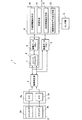••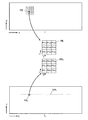•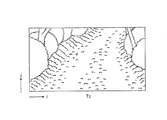•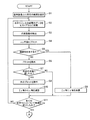•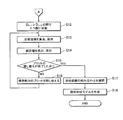•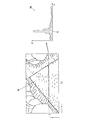•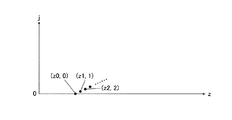•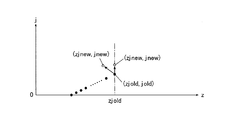•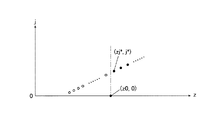•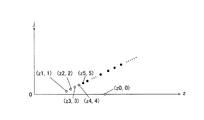•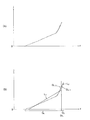•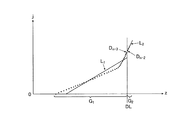•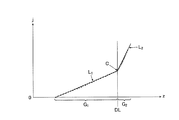•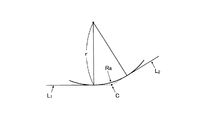•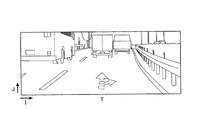•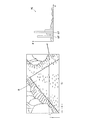•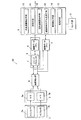•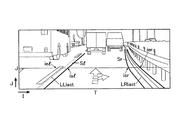•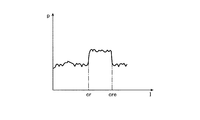•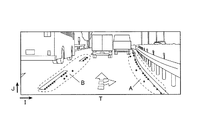•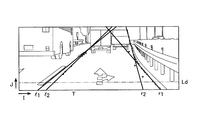•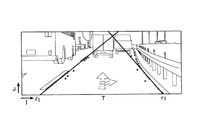•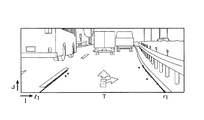•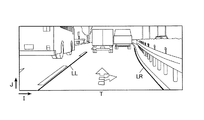•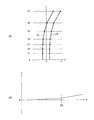## Abstract

The present invention provides a road surface shape recognition device capable of accurately detecting an actual road surface shape even when a lane is marked on a road surface as well as when a lane is not marked.
A road surface shape recognition device 1 detects distance data on a road surface on which a host vehicle travels to generate a distance image Tz, and a distance z on each horizontal line j of the distance image Tz. The representative distance detecting means 10 for voting the data H of the above to the histogram Hj to detect the representative distance zj for each horizontal line j and plotting the representative distance zj on the virtual plane for each horizontal line j, and evaluating that there is no continuity Evaluation means 11 that excludes the plotted plots from the virtual plane, approximate straight line calculation means 12 that calculates approximate straight lines L 1 and L 2 for all plots that remain on the virtual plane without being excluded, and approximation Road surface shape model generation means 13 for generating a road surface shape model using a combination of straight lines L 1 and L 2 .
[Selection] Figure 1

## Description

The present invention relates to a road surface shape recognition device, and more particularly to a road surface shape recognition device that generates a road surface shape model based on position data obtained by a distance image generation means.

In recent years, an imaging device such as a CCD (Charge Coupled Device) camera or a CMOS (Complementary Metal Oxide Semiconductor) camera or a laser radar distance measuring device has been mounted on a vehicle. Development of a road surface shape recognition device for recognizing a road surface shape on which a vehicle equipped with such a device travels is performed by analyzing reflected light of emitted radio waves or laser beams (see, for example, Patent Documents 1 and 2). .

In Patent Document 1, out of a pair of images captured by an imaging device including a stereo camera, each pixel corresponding to a lane on the side of the host vehicle is detected from the image based on the luminance of each pixel of one image. A technique for calculating a model of a road surface shape in the horizontal direction and the vertical direction by linearly approximating the position data of each pixel corresponding to the detected lane for each section set in front of the host vehicle is disclosed. The lane refers to a continuous line or a broken line marked on a road surface such as an overtaking prohibition line or a lane marking that divides a roadside zone and a roadway.

Patent Document 2 discloses a technique for projecting position data obtained by measuring a distance to an object onto three-dimensional coordinates and determining a road surface based on the distribution thereof.

JP 2001-92970 A Japanese Patent Laid-Open No. 10-143659

However, in the technique described in Patent Document 1, it is possible to detect a lane on a normal road in which a lane is marked on the road surface, and to detect a road surface shape based on the detected lane. The shape could not be detected on the road surface where no lane was marked on the road surface.

Moreover, in the technique described in Patent Document 2, it is necessary that the position data detected using a stereo camera or the like is accurate, but in the stereo matching process for a pair of images captured by the stereo camera, There are many cases where mismatches occur. Since position data varies, it is not always easy to determine a road surface from a three-dimensional distribution of position data.

Moreover, in the technique described in Patent Document 2, the effectiveness can be expected on a road surface with many textures such as an unpaved road or a mountain road, but when the texture is relatively small like a normal road, The number of data points of the position data corresponding to the road surface is reduced. If there are other three-dimensional objects or the like, data at a few positions corresponding to the road surface are mixed with data at positions corresponding to the three-dimensional object, and there is a possibility that the road surface shape cannot be detected stably.

The present invention has been made in view of the circumstances as described above, and it is possible to accurately detect the actual road surface shape. Of course, when the lane is marked on the road surface, the lane is marked. An object of the present invention is to provide a road surface shape recognition device capable of accurately detecting the road surface shape even when the road surface is not.

In order to solve the above problem, the first invention is a road surface shape recognition apparatus,
A position image including a horizontal position, height and distance in real space on a road surface on which the vehicle travels is detected at a plurality of different points, and a distance image representing the data of each position on a two-dimensional plane is obtained. A distance image generating means for generating;
The distance data in the position data existing on each horizontal line of the distance image is voted for a histogram created for each horizontal line, and a statistical value in each histogram is calculated for each horizontal line. Representative distance detecting means for detecting as a representative distance and plotting the representative distance on a virtual plane for each horizontal line;
Evaluation means for evaluating the continuity of the plot of the representative distance on the virtual plane for each horizontal line and excluding the plot evaluated as having no continuity from the virtual plane;
Approximating straight line calculating means for calculating approximating straight lines for all the plots remaining on the virtual plane without being excluded by the evaluating means;
Road surface shape model generating means for generating a road surface shape model using a combination of the approximate straight lines;
It is characterized by providing.

According to a second aspect of the present invention, in the road surface shape recognition device according to the first aspect, the representative distance detecting means uses the class value of the class to which the mode value of the frequency of the histogram belongs as a statistical value in the histogram. To do.

According to a third aspect of the present invention, in the road surface shape recognition device according to the first aspect, the representative distance detecting means uses a class value of a class to which a predetermined number of peak values in the frequency of the histogram belong as a statistical value in the histogram, and A class value of a class to which the number of peak values belong is detected as a representative distance of the horizontal line.

4th invention is the road surface shape recognition apparatus in any one of 1st to 3rd invention,
The representative distance detecting means performs processing in order from the lower horizontal line while shifting the horizontal line to be processed upward on the distance image generated by the distance image generating means,
When the representative distance plotted later on the virtual plane is not on the far side of the representative distance plotted earlier, the subsequent plot has continuity with the previous plot. It is evaluated that it does not have, and the subsequent plot is excluded from the virtual plane.

According to a fifth aspect of the present invention, in the road surface shape recognition device according to the fourth aspect of the invention, when the evaluation means reaches a predetermined number of horizontal lines obtained by continuously performing the process of excluding the plot, It is characterized by excluding the plot.

A sixth aspect of the present invention is the road surface shape recognition device according to any one of the first to fifth aspects of the invention, wherein the representative distance detecting means includes past data among the position data existing on each horizontal line of the distance image. Only the data of the distance in the data of the position existing within a predetermined range from the position of the road surface in the current sampling period estimated based on the road surface shape model generated in the sampling period and the subsequent behavior of the host vehicle Are voted on the histogram.

A seventh aspect of the present invention is the road surface shape recognition device according to any one of the first to sixth aspects, wherein the representative distance detecting means includes a traveling path of the host vehicle estimated from the behavior of the host vehicle in the distance image. A predetermined range is set, and only the distance data in the position data existing on each horizontal line within the predetermined range is voted on the histogram.

An eighth invention is the road surface shape recognition device according to any one of the first to seventh inventions,
Lane detection means for detecting a lane on the distance image,
The representative distance detection means sets a predetermined range based on the lane detected by the lane detection means among the horizontal lines of the distance image, and exists on each horizontal line within the predetermined range. Only the distance data in the position data to be voted on the histogram.

A ninth aspect of the invention is the road surface shape recognition device according to any one of the first to eighth aspects of the invention,
The approximate straight line calculation means, for all the plots that remain on the virtual plane without being excluded by the evaluation means, based on the representative distance, the plots on the side closer to the host vehicle. Each time the plot of the boundary part of two groups is transferred from one group to the other group, an approximate straight line that approximates each plot for each group is divided into a group and a group on the far side. Respectively,
The road surface shape model generation means calculates a statistical value based on the approximate line for each group in which the plot is transferred, and based on the calculated statistical value based on the approximate lines of the two groups. One of the combinations of the approximate lines in the two groups is selected, and the road surface shape model is generated using the selected combination of the approximate lines.

A tenth aspect of the invention is the road surface shape recognition device of the ninth aspect of the invention,
The approximate straight line calculating means calculates the approximate straight line that approximates each plot for each group by a least square method,
The road surface shape model generation means calculates, for each group, a variance or a standard deviation of the plots belonging to each group with respect to the approximate line as a statistical value based on the approximate line, and the calculated two groups of the two groups A combination of the approximate lines that minimizes a total value of statistical values based on the approximate lines is selected.

An eleventh aspect of the present invention is the road surface shape recognition device according to the tenth aspect of the invention, wherein the approximate straight line calculating means represents the representative distance as z and the position of the horizontal line in the distance image as j, and the boundary portion to be transferred. When the representative distance in the plot is represented by za, the position of the horizontal line is represented by ja, and the sum for each group is represented by Σ, the plot of the boundary portion is expressed from one group to the other group. each time transferring the, Shigumaz in said one group, Σj, Σz 2, respectively, from Σzj za, ja, subtracts za 2, zaja, Σz in the other group, Σj, Σz 2, respectively Σzj za, The approximate straight lines that approximate the plot for each group are calculated by adding the values of ja, za 2 , and zaja, respectively, by the least square method.

A twelfth aspect of the invention is the road surface shape recognition device according to any one of the first to eighth aspects of the invention, wherein the road surface shape model generation means is not excluded by the evaluation means and remains on the virtual plane. Hough transform is performed on the plot, two approximate straight lines are calculated, and the road surface shape model is generated as a shape on at least the virtual plane.

In a thirteenth aspect of the present invention, in the road surface shape recognition device according to any one of the first to twelfth aspects, the road surface shape model generation means calculates the intersection of the approximate lines of the selected two groups. The generated road surface shape model is corrected by replacing the approximate straight line with a relaxation curve having a tangent line.

A fourteenth aspect of the invention is the road surface shape recognition device according to any one of the first to thirteenth aspects of the invention,
Imaging means for capturing an image of the front of the host vehicle and acquiring an image;
A search is made on a horizontal line on the image to detect a pixel whose luminance difference between adjacent pixels is not less than a predetermined threshold as a lane candidate point, and the horizontal line to be searched is shifted in the vertical direction of the image while shifting the horizontal line. Lanes that detect lane candidate points, connect the detected lane candidate points, detect lanes on the image, and generate a road surface shape model as a shape on at least a virtual plane based on the detected lane information Detection means;
A processing unit that evaluates each of the road surface shape model generated by the road surface shape model generation unit and the road surface shape model generated by the lane detection unit, and selects any one of the road surface shape models;
It is characterized by providing.

A fifteenth aspect of the invention is the road surface shape recognition device according to any one of the first to thirteenth aspects of the invention.
Imaging means for capturing an image of the front of the host vehicle and acquiring an image;
A search is made on a horizontal line on the image to detect a pixel whose luminance difference between adjacent pixels is not less than a predetermined threshold as a lane candidate point, and the horizontal line to be searched is shifted in the vertical direction of the image while shifting the horizontal line. Lanes that detect lane candidate points, connect the detected lane candidate points, detect lanes on the image, and generate a road surface shape model as a shape on at least a virtual plane based on the detected lane information Detection means;
The road surface shape model generated by the road surface shape model generation unit and the road surface shape model generated by the lane detection unit are each evaluated, and a weighted average of both the road surface shape models is generated to generate a road surface shape model. Processing means;
It is characterized by providing.

A sixteenth aspect of the present invention is the road surface shape recognition device according to the fourteenth or fifteenth aspect, wherein the processing means is the road surface shape model generated by the road surface shape model generating means and the road surface shape generated by the lane detecting means. Model, the number of points of data used when detecting each road surface shape model, the range in which the data is detected, each road surface shape model generated in the past sampling period, and the behavior of the host vehicle thereafter. Of the difference between each position of the road surface and the position of each road surface shape model in the current sampling period estimated based on the variance or standard deviation of the data with respect to each approximate line constituting each road surface shape model It is characterized by evaluating each based on at least one of these.

According to the first invention, the distance data of each pixel on each horizontal line of the distance image is voted on the histogram for each horizontal line, and the statistical value is detected as the representative distance for each horizontal line. Is plotted on the virtual plane for each horizontal line, excluding plots that do not have continuity, and an approximation line is calculated for all remaining plots on the virtual plane to determine the most appropriate approximation line. A combination is selected to generate a road surface shape model. Therefore, for example, as shown in FIG. 2 to be described later, it is possible to accurately and stably detect the shape of a road surface on which a lane is not marked on a road surface such as an unpaved road or a mountain road.

Even if a mismatch occurs in stereo matching processing or abnormal data is obtained by a laser radar ranging device, the frequency of occurrence of such data on each horizontal line is smaller than the frequency of normal values. Therefore, such data is shaken off in the voting process for each histogram of the distance data of each pixel on each horizontal line of the distance image. Even if such data is adopted as the representative distance, the plot is excluded if the corresponding plot does not have continuity. Therefore, even when a mismatch occurs in the stereo matching process or abnormal data is obtained by the laser radar distance measuring device, the road surface shape can be accurately and stably detected.

Further, on a road surface on which a lane or the like is marked, for example, data of a relatively large number of distances are effectively detected at the edge portion of the marking such as a lane illustrated in FIG. 16 to be described later or an arrow marked on the road surface. Therefore, it becomes possible to accurately and stably detect the road surface shape based on it.

According to the second invention, in addition to the effect of the invention, the class value of the class to which the mode value of the frequency of the histogram belongs is used as the statistical value in the histogram, so that the distance that appears most frequently on the horizontal line. Is detected as a representative distance of the horizontal line, and a road surface shape model can be accurately generated based on the detected value.

According to the third invention, in addition to the effect of the invention, the class value of the class to which the predetermined number of peak values in the frequency of the histogram belongs is used as the statistical value in the histogram, so that the distance that appears frequently on the horizontal line can be reduced. Each class value is detected as a representative distance of the horizontal line, and a road surface shape model can be accurately generated based on these.

According to the fourth aspect of the present invention, in the road surface shape, the lower horizontal line of the distance image should detect the representative distance closer to the host vehicle than the upper horizontal line. Therefore, by performing the processing in the representative distance detection means in order from the horizontal line on the lower side of the distance image, when plotting them on the virtual plane, the representative distance of the plot plotted later is the same as that of the plot plotted earlier. If it is not on the far side from the representative distance, it is possible to easily and accurately evaluate the subsequent plot if it does not have continuity with the previous plot.

Therefore, in such a case, by configuring the subsequent plot to be excluded from the virtual plane, it becomes possible to accurately exclude a plot that is not suitable as a road surface shape, and to generate a road surface shape model. The effect of the invention can be exhibited more accurately, and the reliability of the generated road surface shape model can be further improved.

According to the fifth invention, the subsequent plots are excluded in the fourth invention, but if the previous plots were originally plotted based on an abnormal representative distance, the subsequent normal plots were successively It is inconvenient because it is excluded. Therefore, when the number of horizontal lines that have been continuously excluded from the plot reaches a predetermined number, the previous plot is excluded, so that the abnormal previous plot is excluded and normal It is possible to make a simple plot as a target for generating a road surface shape model, and to exhibit the effect of the fourth invention more accurately.

According to the sixth aspect of the present invention, the distance existing within a predetermined range from the road surface position in the current sampling cycle estimated based on the road surface shape model generated in the past sampling cycle and the subsequent behavior of the host vehicle. By using only data as a target for voting on the histogram, the effects of the above-described inventions can be exhibited accurately, and a road surface shape model can be stably generated for each sampling period.

According to the seventh invention, in addition to the effects of the respective inventions, the search range of the data of the position existing on each horizontal line is set to a predetermined range including the traveling path of the host vehicle where the road surface is highly likely to exist. By limiting the speed, it is possible to speed up the processing, and it is possible to generate a road surface shape model without being affected by three-dimensional objects outside the traveling road, and stably generate the road surface shape model. It becomes possible to do.

According to the eighth invention, in addition to the effects of the respective inventions, in the case where the apparatus includes a lane detection means, it is highly possible that a road surface exists in a search range of data at positions on each horizontal line. By limiting to a predetermined range including the lane, it is possible to speed up the processing, and it is possible to generate a road surface shape model without being affected by a solid object outside the traveling road. A shape model can be generated stably.

According to the ninth invention, in the actual road design, the shape of the road on the virtual plane is constituted by two straight lines, but an appropriate combination of the two approximate straight lines is obtained while changing the group boundary. By searching, in accordance with the actual road design, a road surface shape model can be generated accurately and at high speed by combining two approximate straight lines, and the road surface shape can be recognized accurately. . Further, by generating a road surface shape model using a combination of approximate lines selected from a combination of two groups of approximate lines based on statistical values based on the approximate lines, the reliability of the generated road surface shape model can be improved. It becomes possible to improve more and the effect of said each invention is exhibited more correctly.

According to the tenth invention, in the ninth invention, an approximate line for approximating each plot for each group is calculated by the least square method, and a statistical value based on the approximate line is calculated for the approximate line of each plot belonging to each group. By calculating as the total value of the variances or standard deviations, it is possible to accurately and easily select a combination of approximate straight lines, and to exhibit the effects of the ninth invention more accurately.

According to the eleventh invention, in the tenth invention, each time the distance data Da of the boundary portion of each group is transferred from one group to the other group when the approximate straight line is calculated using the least square method. Since it is possible to calculate each equation of the approximate line simply by subtracting za corresponding to the distance data Da from each sum Σz etc. in one group and adding it to each sum Σz etc. in the other group. The approximate straight line can be calculated easily and at high speed, and the effects of the tenth aspect of the invention can be exhibited more accurately.

According to the twelfth aspect, the combination of approximate straight lines can be obtained by the Hough transform for all plots remaining on the virtual plane without being excluded by the evaluation means. Therefore, by calculating two approximate straight lines by Hough transform and generating a road surface shape model, a road surface shape model can be generated by selecting a combination of approximate straight lines easily and accurately. It is possible to exert the effect accurately.

According to the thirteenth invention, in the actual road design, the shape of the road on the virtual plane is composed of two straight lines and a relaxation curve. In accordance with the actual road design, the road surface shape model is It is possible to accurately generate a combination of two approximate straight lines and a relaxation curve, and it is possible to accurately recognize the road surface shape. Therefore, the effects of the above inventions can be more accurately exhibited.

According to the sixteenth aspect of the present invention, the two road surface shape models are divided into the number of data points used when detecting each road surface shape model, the range in which the data is detected, and each road surface generated in the past sampling period. The difference between each position of the road surface and the position of each road surface shape model in the current sampling cycle estimated based on the shape model and the behavior of the host vehicle thereafter, the distribution of data for each approximate line constituting each road surface shape model, or By evaluating each based on at least one of the standard deviations, a more reliable road surface shape model is selected or a new road surface shape model is generated by weighted averaging of both road surface shape models according to the evaluation. It becomes possible, and it becomes possible to exhibit the effect of each said invention more correctly.

It is a block diagram which shows the structure of the road surface shape recognition apparatus which concerns on 1st Embodiment. It is a figure which shows the example of the reference | standard image imaged with an imaging means. It is a figure explaining the principle of stereo matching. It is a figure which shows the example of the produced distance image. It is a flowchart which shows the procedure of each process in a process part. It is a flowchart which shows the procedure of each process in a process part. It is a figure explaining the histogram etc. which are produced for every predetermined range and horizontal line which are set in a distance image. It is a figure explaining the example of the representative distance plotted on the virtual plane (zj plane). It is a figure explaining the example of the plot which does not exist in the far side rather than the representative distance plotted previously. It is a figure explaining the example of the plot after being excluded by the abnormal previous plot. It is a figure explaining that a previous plot is excluded when the process which excludes the subsequent plot in the plot of FIG. 10 is performed continuously. (A) It is a figure explaining the example of each plot which remain | survives on a virtual plane, (B) The boundary set between the plot whose representative distance is the 2nd most distant and the 3rd most distant plot in (A) FIG. It is a figure explaining the state which changed the plot of the boundary part, and set the boundary between the plot whose representative distance is the 3rd farthest and the 4th farthest. It is a figure showing the combination of the selected approximate line, a boundary, an intersection, etc. It is a figure showing the circular arc which is a relaxation curve which makes each approximate straight line a tangent. It is a figure which shows the example of the reference | standard image by which the lane etc. were imaged. It is a figure explaining the state where two peak values appeared in the frequency of the histogram in FIG. It is a block diagram which shows the structure of the road surface shape recognition apparatus which concerns on 2nd Embodiment. It is a figure explaining a horizontal line, a search area | region, a search start point, a search end point, etc. It is a graph showing the brightness | luminance of each pixel obtained by searching on a horizontal line. It is a figure showing the lane candidate point detected on the reference | standard image. It is a figure showing the straight line extracted as a result of a Hough conversion process. It is a figure showing the straight line selected as a straight line suitable for a lane from each straight line of FIG. It is a figure showing the straight line decided on the near side of the own vehicle. It is a figure showing the left and right lane detected on the reference | standard image. It is a figure which shows the example of the road surface model produced | generated by the lane detection means, (A) represents a horizontal shape model, (B) represents a road height model, ie, a road surface shape model.

Embodiments of a road surface shape recognition apparatus according to the present invention will be described below with reference to the drawings.

[First Embodiment]
As shown in FIG. 1, the road surface shape recognition apparatus 1 according to the first exemplary embodiment of the present invention includes an imaging unit 2, a distance image generation unit 6, a representative distance detection unit 10, an evaluation unit 11, an approximate straight line calculation unit 12, A processing unit 9 having a road surface shape model generation unit 13 and the like is provided.

The upstream configuration of the processing unit 9 including the distance image generating means 6 and the like is described in detail in the Patent Document 1 and the like previously filed by the applicant of the present application, and a detailed description of the configuration is given in the above. Leave it to the gazette. A brief description is given below.

In the present embodiment, the image pickup means 2 includes a built-in image sensor such as a CCD or a CMOS sensor that is synchronized with each other, and is mounted in the vicinity of a vehicle rearview mirror, for example, at a predetermined interval in the vehicle width direction. This is a stereo camera composed of a main camera 2a on the passenger side and a sub camera 2b on the passenger seat side, and is configured to capture a predetermined sampling period and output a pair of images.

In the following, one image as shown in FIG. 2 captured by the main camera 2a is referred to as a reference image T, and an image (not shown) captured by the sub camera 2b is referred to as a comparative image Tc. In the following, the case where the process in the approximate straight line calculation unit 12 or the like is performed on the reference image T will be described. However, the process is performed on the comparison image Tc or both the images T and Tc are processed. It is also possible.

In the present embodiment, the main camera 2a and the sub camera 2b of the imaging unit 2 each acquire monochrome image data. However, color image data represented by RGB values or the like is captured. It is also possible to use an imaging means, and the present invention is also applied to such a case.

When the reference image T or the like is picked up by the main camera 2a or the like, for example, as shown in FIG. 2, the image pickup starts from the leftmost pixel of each horizontal line J such as the reference image T and then sequentially scans in the right direction. To go. Further, the image data of the luminance of each pixel such as the reference image T is converted into the conversion means 3 in such a manner that the horizontal line J to be scanned is picked up while switching upward from the lowermost line in order. Are sent sequentially.

The conversion unit 3 includes a pair of A / D converters 3a and 3b, and each image data for each pixel such as the reference image T captured by the main camera 2a and the sub camera 2b of the imaging unit 2 is 256, for example. The image data is sequentially converted into digital image data as the gray scale luminance of the gradation and output to the image correction unit 4.

In addition, the image correction unit 4 sequentially performs image correction such as displacement and noise removal, luminance correction, etc. on each image data, and stores each image data in the image data memory 5 sequentially, and also in the processing unit 9. It is designed to transmit sequentially. Further, the image correction unit 4 sequentially transmits the image data of the reference image T and the comparison image Tc subjected to the image correction to the distance image generation unit 6.

The distance image generating means 6 includes an image processor 7 and detects data of a position including a horizontal position x, a height y and a distance z in the real space on a road surface on which the host vehicle travels at a plurality of different points. In addition, a distance image Tz in which data at each position is represented on a two-dimensional plane is generated.

The image processor 7 of the distance image generating means 6 sequentially performs stereo matching and filtering processing on the image data of the reference image T and the comparison image Tc so as to sequentially calculate the parallax dp for each pixel of the reference image T. It has become.

In stereo matching performed by the image processor 7, as shown in FIG. 3, the reference image T is divided into reference pixel blocks PB having a predetermined number of pixels such as 3 × 3 pixels and 4 × 4 pixels. Then, for each reference pixel block PB, each comparison pixel block PBc on the epipolar line EPL having the same J coordinate as that of the reference pixel block PB in the comparison image Tc is searched for.
SAD = Σ | p1st−p2st | (1)
And the comparison pixel block PBc having the smallest SAD value is specified.

In Equation (1), p1st represents the luminance of each pixel in the reference pixel block PB, and p2st represents the luminance of each pixel in the comparison pixel block PBc. Further, the above sum is obtained when the reference pixel block PB and the comparison pixel block PBc are set as a 3 × 3 pixel region, for example, a range of 1 ≦ s ≦ 3, 1 ≦ t ≦ 3, and 4 × 4 pixels. When set as a region, calculation is performed for all pixels in the range of 1 ≦ s ≦ 4 and 1 ≦ t ≦ 4.

In stereo matching, the parallax dp is sequentially obtained for each pixel of the reference image T from the position on the reference image T of the reference pixel block PB and the position on the comparison image Tc of the comparison pixel block PBc specified for the reference pixel block PB. It is calculated.

In real space, the point on the road surface directly below the center of the pair of cameras 2a, 2b is the origin, the vehicle width direction (ie, horizontal direction) of the host vehicle is the X-axis direction, and the vehicle height direction (ie, height direction). ) In the Y-axis direction and the vehicle length direction (that is, the distance direction) as the Z-axis direction, position data (x, y, z) including the horizontal position x, height y, and distance z in real space; The pixel coordinates (I, J) and the parallax dp on the reference image T can be associated one-to-one by coordinate conversion based on the principle of triangulation expressed by the following equations (2) to (4).
x = CD / 2 + z * PW * (I-IV) (2)
y = CH + z × PW × (J−JV) (3)
z = CD / (PW × (dp−DP)) (4)

In the above equations, CD is the distance between the pair of cameras, PW is the viewing angle per pixel, CH is the mounting height of the pair of cameras, and IV and JV are I on the reference image T at the infinity point in front of the host vehicle. Coordinates, J coordinates, and DP represent vanishing point parallax.

In the filtering process, the image processor 7 outputs the calculated parallax dp as invalid when the degree of similarity of the luminance pattern between the reference pixel block of the reference image T and the pixel block of the comparison image Tc is low. It has become.

For this reason, in the present embodiment, the distance image generation unit 6 uses only the parallax dp calculated as valid by the image processor 7 among the parallaxes dp calculated for each pixel of the reference image T in this way (4 ), The calculated distance z in the real space is assigned to each corresponding pixel (I, J) of the reference image T, and the data at each position is placed on a two-dimensional plane as shown in FIG. The represented distance image Tz is generated.

As described above, in stereo matching, one parallax dp is assigned to a reference pixel block PB of, for example, 3 × 3 pixels on the reference image T. Therefore, as described above, 3 × 3 pixels on the reference image T. The same parallax dp is assigned to these nine pixels.

In the following process, the distance image Tz is generated so that one reference pixel block PB of 3 × 3 pixels, for example, on the reference image T is regarded as one pixel of the distance image Tz.

In this case, the coordinates (i, j) of each pixel of the distance image Tz and the coordinates (I, J) of each pixel of the reference image T are different, but the coordinates (i, j) of each pixel of the distance image Tz are different. ) And the parallax dp and the horizontal position x, height y, and distance z in the real space are associated one-to-one according to the following equations (5) to (7) similar to the above equations (2) to (4). be able to.
x = CD / 2 + z * PW * (i-IV) (5)
y = CH + z * PW * (j-JV) (6)
z = CD / (PW × (dp−DP)) (7)

In this case, the meanings of CD, PW, CH, IV, JV, and DP are the same as those in the above formulas (2) to (4), but each numerical value is replaced for the distance image Tz. The coordinates (i, j) and parallax dp of each pixel of the distance image Tz, and the horizontal position x, height y, and distance z in the real space are in a one-to-one relationship according to the expressions (5) to (7). Is associated with.

Further, instead of generating a distance image Tz by assigning a real space distance z to each pixel of the reference image T as in the present embodiment, a valid parallax dp is assigned to each pixel of the reference image T and the distance image is generated. It can also be configured to generate Tz.

The distance image generation means 6 stores the distance image Tz generated in this way in the distance data memory 8 and sequentially transmits it to the processing unit 9.

In the present embodiment, as described above, the distance image generation unit 6 calculates the distance z in the real space calculated by stereo matching or the like with respect to the reference image T and the comparison image Tc captured by the main camera 2a and the sub camera 2b. Although the case where it is comprised so that the distance image Tz may be produced | generated based on parallax dp is demonstrated, it is not limited to this.

That is, the distance image generation means 6 can detect data of positions including the horizontal position x, height y, and distance z in real space on a road surface on which the host vehicle travels at a plurality of points different from each other. It suffices if the distance image Tz can be generated by representing the data of each position on a two-dimensional plane. For example, like the laser radar distance measuring device described above, it is possible to irradiate a laser beam in front of the host vehicle and detect position data on the road surface on which the host vehicle travels based on information of the reflected light at a plurality of points. Any detection method can be used, and the detection method is not limited to a specific method.

In this embodiment, the processing unit 9 is configured by a computer in which a CPU (Central Processing Unit), a ROM (Read Only Memory), a RAM (Random Access Memory), an input / output interface, and the like (not shown) are connected to a bus. The processing unit 9 includes a representative distance detection unit 10, an evaluation unit 11, an approximate straight line calculation unit 12, a road surface shape model generation unit 13, and a memory (not shown).

Note that the processing unit 9 may be configured to perform other processing such as object detection for detecting an object such as a preceding vehicle. The processing unit 9 is connected to sensors Q such as a vehicle speed sensor, a yaw rate sensor, and a steering angle sensor for measuring the steering angle of the steering wheel. Measurement values such as a vehicle speed, a yaw rate, and a steering angle are input as needed. It has become so.

Hereinafter, each process in the process part 9 is demonstrated according to the flowchart of FIG. 5 and FIG. 6, and the effect | action of the road surface shape recognition apparatus 1 which concerns on this embodiment is demonstrated.

In the present embodiment, the representative distance detection unit 10 first calculates a travel path of the host vehicle that is estimated to travel in the future from the behavior of the host vehicle, that is, the vehicle speed and yaw rate of the host vehicle, in the distance image Tz. As shown in FIG. 7, a predetermined range R including the calculated traveling path is set on the distance image Tz (step S1). The predetermined range R need not always be set.

Then, the representative distance detecting means 10 subsequently votes the data of the distance z in the real space existing on each horizontal line j of the distance image Tz to the histogram Hj created for each horizontal line j. (Step S2). At this time, in the present embodiment, the representative distance detecting unit 10 votes only the data of the distance z existing on the horizontal line j within the predetermined range R to the histogram Hj. This is because the data of the distance z in the real space other than the predetermined range R including the traveling path of the host vehicle is not necessarily required for detecting the road surface shape, and is intended to speed up the processing by limiting the processing range. .

Further, in this embodiment, the representative distance detecting means 10 is a case where the position data existing on each horizontal line j, that is, the distance z in the position data is plotted on the virtual plane for each horizontal line j. The plot (z, j) exists within a predetermined range from the road surface position in the current sampling period estimated based on the road surface shape model (described later) generated in the past sampling period and the behavior of the host vehicle thereafter. Only data of such a distance z is voted on the histogram Hj. This is because data of a position that is far away from the position of the road surface estimated from a past road surface shape model or the like is not suitable for detection of the road surface shape.

Further, as described above, in this embodiment, image data is transmitted from the imaging unit 2 in order from the lowest horizontal line j of the reference image T, and the real space of the distance image Tz generated by the distance image generation unit 6. The data of the upper distance z is also input in order from the lowermost horizontal line j. Therefore, the representative distance detection means 10 also votes the data of the distance z in the real space in order from the lower horizontal line j in the distance image Tz to the histogram Hj, and when the voting on the lower horizontal line j ends, The data of the distance z in the real space of the horizontal line j + 1 immediately above is voted for the histogram Hj + 1, and the data of the distance z in the real space is voted for each histogram Hj for each horizontal line j.

In this embodiment, the representative distance detecting unit 10 shifts the horizontal line j to be processed on the distance image Tz generated by the distance image generating unit 6 in this way from the lower horizontal line j. Processing is performed in order.

When the representative distance detection unit 10 completes voting to the histogram Hj of the data of the distance z in the real space existing within the predetermined range R of the horizontal line j in this way, the representative distance detection unit 10 in the histogram Hj of the horizontal line j A statistical value is calculated, and the statistical value is detected as a representative distance zj of the horizontal line j (step S3).

In the present embodiment, as shown in FIG. 7, the representative distance detection means 10 uses the class value zj of the class to which the mode value of the frequency F of the histogram Hj belongs as a statistical value in the histogram Hj. The representative distance detecting means 10 detects the class value zj of the class to which the mode value of the frequency F belongs as the representative distance zj of the horizontal line j.

Further, as shown in FIG. 8, the representative distance detecting means 10 plots the representative distance zj of the detected horizontal line j on a virtual plane (hereinafter referred to as zj plane) for each horizontal line j. (Step S4).

Further, as described above, in this embodiment, the representative distance detection unit 10 shifts the horizontal line j to be processed upward, and performs plotting processing of the representative distance zj on the zj plane for each horizontal line j. To do.

The evaluation means 11 evaluates the continuity of the plot of the representative distance z on the zj plane performed by the representative distance detection means 10 for each horizontal line j (step S5), and has continuity. The plot evaluated as not (step S5; NO) is excluded from the zj plane (step S6). As described above, there are not a few cases in which mismatching occurs in stereo matching processing, and if the plot does not have continuity corresponding to the road surface, the plot is not suitable for detection of the road surface shape.

In the present embodiment, when the representative distance zjnew plotted later on the zj plane is not on the far side of the representative distance zjold plotted earlier, the evaluation unit 11 performs the subsequent plotting first. Therefore, the subsequent plot is excluded from the zj plane.

Specifically, as shown in FIG. 9, the representative distance zjnew plotted later is closer to the representative distance zjold plotted earlier, or the representative distance zjold plotted earlier is used. Existing at the same distance zjold in the real space as the road surface shape is abnormal. Therefore, in such a case, the evaluation unit 11 evaluates the subsequent plot (zjnew, jnew) as not having continuity with the previous plot (zjold, jold), and the subsequent plot (zjnew). , Jnew) are excluded from the zj plane (step S6).

On the other hand, as shown in FIG. 10, for example, when an abnormal representative distance z0 is detected by the representative distance detecting means 10 due to a large number of mismatches occurring in the first horizontal line j (j = 0) or the like, Even if the normal representative distance zj is detected in the horizontal line j, if the above principle is followed, all plots having the normal representative distance zj are excluded, and the representative distance zj farther than the representative distance z0 is excluded. The plot on the zj plane is finally resumed when a plot (zj * , j * ) having * appears.

Further, in this state, as can be seen from FIG. 10, for example, the inclination of the plot (z0, 0) and the plot (zj * , j * ) with respect to the Z-axis direction and the plot (zj * , j * ) It is difficult to say that the slope continuity with respect to the Z-axis direction of each plot is the same slope, and it is difficult to say that the continuity of the plot representing the road surface shape is ensured.

Therefore, in this embodiment, the evaluation unit 11 sets the horizontal line j in such a case, that is, the number of horizontal lines j obtained by continuously performing the process of excluding the plot by evaluating as not having continuity, for example, to 4 or the like. When the predetermined number is reached (step S7; YES), the previous plot is excluded (step S8).

Therefore, for example, when the situation shown in FIG. 10 occurs, the evaluation unit 11 makes a plot (z0,0) corresponding to the horizontal line j (j = 0) on the zj plane as shown in FIG. ) Are plotted, and each plot corresponding to the horizontal lines j (j = 1), j (j = 2), j (j = 3), j (j = 4) is evaluated as having no continuity. When the processing of excluding z1,1), (z2,2), (z3,3), and (z4,4) is continuously performed, the plot (z0) corresponding to the horizontal line j (j = 0) , 0) is an abnormal plot, the plot (z0, 0) is excluded from the zj plane. In this case, the evaluation unit 11 restarts plotting on the zj plane from the plot (z5, 5) corresponding to the horizontal line j (j = 5).

The approximate straight line calculation means 12 calculates approximate straight lines for all plots (zj, j) remaining on the zj plane (virtual plane) without being excluded by the evaluation means 11. . Since there is also a plot (zj, j) excluded by the evaluation means 11 as described above, in the following, the coordinates of the plot are simply expressed as (z, j), and the above representative distance zj is simply expressed as the representative distance z. .

Hereinafter, first, an outline of processing in the approximate straight line calculation means 12 will be described.

In the present embodiment, the approximate straight line calculation unit 12 determines all the plots (z, j) that are not excluded by the evaluation unit 11 and remain on the zj plane based on the representative distance z. the plot and divides the side into the group G 1 and the farther the group of the side G 2 near the vehicle. Each time the plot of the boundary portion between the two groups G 1 and G 2 is transferred from one group to the other group, approximate lines L 1 and L 2 that approximate the plots belonging to the groups G 1 and G 2 are approximated. Are calculated for each of the groups G 1 and G 2 .

Further, in the present embodiment, approximation line calculating means 12, using the least squares method, for each group G 1, G 2, the approximate straight line L 1 which approximates the each plot belonging to each group G 1, G 2 respectively, and calculates the L 2.

Each plot (z, j) is
j = a × z + b (8)
As is well known, in the least square method, a and b are as follows.
a = (nΣzj−Σz · Σj) / {nΣz 2 − (Σz) 2 } (9)
b = (Σz 2 · Σj−Σzj · Σz) / {nΣz 2 − (Σz) 2 } (10)
It is calculated in the form of In the above equations (9) and (10), n represents the number of plots in each group, and the total sum Σ is performed for all plots in each group.

In the present embodiment, the division of the groups G 1 and G 2 and the calculation of the approximate lines L 1 and L 2 in the approximate line calculation unit 12 are performed as follows.

It is assumed that each plot that is not excluded by the evaluation unit 11 remains on the zj plane as shown in FIG. In this case, it is assumed that Σz, Σj, Σz 2 and Σzj necessary for the calculations of the above expressions (9) and (10) have already been calculated for all plots.

First, as shown in FIG. 12 (B), the approximate straight line calculation means 12 has a plot D n−1 that is the second most distant plot D and a plot D that is the third furthest from among all remaining plots D. The boundary DL is set between n−2 , and all plots D are automatically composed of n−2 plots D from the plot D 1 having the closest representative distance z to the plot D n−2, which is the third most distant. It is far from the own vehicle (z = 0) consisting of the group G 1 on the side closer to the vehicle (z = 0) and the two plots D, the plot D n−1 having the second most distant representative distance z and the plot D n farthest. divided into groups of side G 2.

Then, for each group G 1, G 2, and calculates an approximate straight line L 1, L 2 that approximates a plurality of plot D belonging to each group G 1, G 2, respectively. That is, in the group G 1, performs linear approximation with respect to the plot D 1 to D n-2 of n-2 pieces of plot D, the group G 2, the two plots D plot D n-1, D n A straight line approximation is performed on the lines to calculate approximate straight lines L 1 and L 2 , respectively.

At this time, for each plot D 1 to D n-2 belonging to the group G 1 , the plots D n−1 and D n of z, j of the group G 2 from the already calculated Σz, Σj, Σz 2 and Σzj. , Z 2 , zj are subtracted, and their values are substituted into the above equations (9) and (10) to calculate a and b, and are substituted into the above equation (8) to obtain the approximate straight line L 1 The equation j = a 1 × z + b 1 is calculated.

For each plot D n−1 , D n belonging to the group G 2 , z, j, z 2 , zj calculated for each plot D n−1 , D n are added, that is, Σz, Σj, Σz 2, calculates the Shigumazj, those values above (9), (10) are substituted into equation a, calculates b, thereof equation (8) is substituted into the approximate straight line L 2 wherein j = a 2 × z + b 2 is calculated.

Subsequently, the plot D n-2 of the boundary DL portion transferred from a group G 1 in the group G 2, the front side That is, as shown in FIG. 13 the position of the boundary DL for dividing the group G 1 and the group G 2 Move one vehicle toward the vehicle (z = 0). That is, the boundary DL is set between the plot D n−2 where the representative distance z is the third furthest and the plot D n−3 which is the fourth furthest.

Then, z n corresponding to the plot D n−2 to be transferred from each sum Σz, Σj, Σz 2 , Σzj for each plot D 1 to D n−2 belonging to the group G 1 calculated as described above. −2 , j n−2 , z n−2 2 , z n−2 j n−2 are subtracted, respectively, and the respective sums Σz, Σj, and the respective sums Σz, Σj for the plots D 1 to D n-3 belonging to the group G 1 Σz 2 and Σzj are calculated.

As for the group G 2, the sum Shigumaz for each plot D n-1, D n which belongs to the group G 2 calculated as described above, Σj, Σz 2, the Shigumazj, plot is transferred D n- 2 by adding the z n-2, j n- 2, z n-2 2, z n-2 j n-2 corresponding, respective for each plot D n-2 to D n which belongs to the group G 2 Sums Σz, Σj, Σz 2 , and Σzj are calculated.

Then, to calculate the equation j = a 1 × z + b 1 of the approximate straight line L 1 for each plot D 1 ~D n-3 belonging to the group G 1 in the same manner as described above, each plot D n-2 belonging to the group G 2 to D n to calculate the equation j = a 2 × z + b 2 of the approximate straight line L 2 on.

In the present embodiment, the approximate straight line calculation means 12 is based on the representative distance z for all plots D remaining on the zj plane (virtual plane) without being excluded by the evaluation means 11 in this way. All the plots D are divided into a group G 1 on the side closer to the host vehicle and a group G 2 on the far side, and the plot Da of the boundary DL portion between the two groups G 1 and G 2 is changed from one group G 1 to the other. Each time the transfer to the group G 2 is performed, za, ja, za 2 and zaja calculated from the plot Da (xa, ja) are subtracted from the respective sums Σz, Σj, Σz 2 and Σzj in the group G 1 , each sum Shigumaz in G 2, Σj, Σz 2, by adding the Shigumazj, has an approximate straight line L 1, L 2 approximating each plot for each group G 1, G 2 to be calculated.

According to this structure, the sum Shigumaz in each group G 1, G 2, Σj, Σz 2, approximate line calculated easily and fast to each Shigumazj, approximating each plot for each group G 1, G 2 L 1 and L 2 can be calculated easily and at high speed, respectively.

In the above configuration example, the position of the boundary DL that divides the group G 1 and the group G 2 is set on the farthest side from the own vehicle, and the approximate straight line L is moved while moving the position of the boundary DL in a direction approaching the own vehicle. 1 and L 2 have been described, but conversely, the position of the boundary DL is closest to the vehicle, that is, the plot D 2 where the representative distance z is second closest and the plot D closest to third. It is also possible to set between 3 and to calculate the approximate lines L 1 and L 2 while moving the position of the boundary DL in a direction away from the host vehicle.

Also in this case, every time the boundary portion plot Da is transferred from the group G 2 to the group G 1 , za, ja, za 2 , zaja corresponding to the plot Da are converted into the sums Σz, Σj, Σz 2 in the group G 1 . It adds to Σzj, Σz the sum of the group G 2, Σj, Σz 2, only subtracted from Shigumazj, the sum Shigumaz in each group G 1, G 2, Σj, Σz 2, Σzj easy and fast to each It is possible to calculate.

Therefore, as shown in the flowchart of FIG. 5, the approximate straight line calculating means 12 first calculates z−, in order to calculate Σz, Σj, Σz 2 , Σzj necessary for the calculations of the above equations (9) and (10). Each time the plot on the j plane is evaluated by the evaluation means 11 as having continuity and plotted without being excluded (step S5; YES), the previously calculated Σz, Σj, Σz 2 , Σzj Z, j, z 2 and zj calculated from the current plot (z, j) are added (step S9).

If the plot on the z-j plane is evaluated as having no continuity by the evaluation unit 11 and excluded (step S8), the approximate straight line calculation unit 12 has already calculated Σz, Σj, Σz 2 , If Σzj has been calculated, z, j, z 2 and zj calculated from the current plot (z, j) are subtracted from Σz, Σj, Σz 2 and Σzj, respectively (step S10).

The representative distance detection unit 10, the evaluation unit 11, and the approximate line calculation unit 12 perform the above processing every time data of the distance z for each horizontal line j of the distance image Tz is transmitted from the distance image generation unit 6. If the processing has not been completed for all horizontal lines j (step S11; NO), the above processing is performed for the next horizontal line j + 1 (that is, the horizontal line j + 1 immediately above the horizontal line j in the present embodiment). It is supposed to repeat.

In the present embodiment, as described above, the predetermined range R (see FIG. 7) is set on the distance image Tz (step S1), and the data of the distance z in the predetermined range R is processed. All the horizontal lines j in the determination process of step S11 indicate horizontal lines j in which a predetermined range R exists.

On the other hand, when the processing is completed for all the horizontal lines j (step S11; YES), the approximate straight line calculation means 12 makes all the plots D remaining on the zj plane (virtual plane) as described above. of, first, the representative distance z plot distant second D n-1 and the third distant plot D n-2 sets the boundary DL between to the group closer to all plots D in the vehicle G 1 and the group G 2 on the far side (step S12 in FIG. 6), approximate lines L 1 and L 2 that approximate the respective plots for each of the groups G 1 and G 2 are calculated, and the group G 1 , G 2 and the information of the approximate lines L 1 , L 2 such as a 1 , b 1 , a 2 , b 2, etc. for each of the groups G 1 and G 2 are stored in the memory in association with each other. (Step S13).

In addition, the road surface shape model generation unit 13 calculates a statistical value based on the approximate lines L 1 and L 2 calculated for each of the groups G 1 and G 2 by the approximate line calculation unit 12 transferring the plot D, and stores the statistical values. (Step S14).

In the present embodiment, the road surface shape model generating unit 13, approximated straight line L 1, L as 2 based statistics, variance sigma 1 for approximation lines L 1, L 2 of each plot D belonging to each group G 1, G 2 2 and σ 2 2 are calculated according to the following equations (11) and (12), respectively.
σ 1 2 = Σ {(a 1 × z + b 1 ) −j} 2 / n 1 (11)
σ 2 2 = Σ {(a 2 × z + b 2 ) −j} 2 / n 2 (12)

Note that n 1 and n 2 represent the numbers of plots D belonging to the groups G 1 and G 2 , respectively. Moreover, as the statistical value, instead of the variance sigma 2, it is also possible to constitute the standard deviation of each plot D belonging to each group G 1, G 2 to calculate for each group G 1, G 2.

If the transfer of the plot Da of the boundary DL portion between the two groups G 1 and G 2 to the group G 2 on the far side from the group G 1 on the far side of the host vehicle has not been completed (step S15; NO) ), a plot Da boundary DL portion transferred from a group G 1 in the group G 2 (step S16), and repeats the processing of steps S13, S14.

When the transfer of the plot Da of the boundary DL portion between the two groups G 1 and G 2 to the group G 2 on the far side from the group G 1 on the far side is completed (step S15; YES), the road surface The shape model generation means 13 is, for example, a total value of σ 1 2 and σ 2 2 based on a statistical value calculated for each combination of the approximate lines L 1 and L 2 among the combinations of the approximate lines L 1 and L 2. There are equal to select a combination of the approximation lines L 1, L 2 having the smallest, two groups G 1, G approximate line L 1 of 2, selects one from among the combinations of L 2 (step S17 ).

Then, the road surface shape model generation means 13 generates a road surface shape model as a shape on the zj plane (virtual plane) using the selected combination of the approximate lines L 1 and L 2 (step S18). ).

For example, in the case of a plot D performed on the zj plane as shown in FIG. 12A, the road surface shape model generation means 13 uses a combination of approximate straight lines L 1 and L 2 as shown in FIG. A road surface shape model is generated as a shape on the zj plane (virtual plane). That is, in this case, the vehicle (z = 0) approximate line L 1 in the range of up to the boundary DL from the distant range than the boundary DL road surface shape model to model an approximate straight line L 2 is generated.

Further, in the present embodiment, the road surface shape model generation unit 13 further approximates each intersection C of the approximate lines L 1 and L 2 of the two groups G 1 and G 2 selected as shown in FIG. The generated road surface shape models L 1 and L 2 are corrected by replacing the straight lines L 1 and L 2 with relaxation curves that are tangents.

In the present embodiment, an arc Ra as shown in FIG. 15 is used as the relaxation curve. It is also possible to use a quadratic curve or the like as the relaxation curve. When the arc Ra is used as the relaxation curve, the radius of curvature r may be a fixed value set in advance, and depends on the difference between the slopes a 1 and a 2 of the approximate straight lines L 1 and L 2 , etc. You may comprise so that it may change. Furthermore, the curvature radius r can be variously changed, and the optimum curvature radius r can be calculated based on the variance or the like of each plotted plot D with respect to the arc Ra.

The road surface shape model generation unit 13 calculates the road surface shape model calculated as described above, that is, information on the selected approximate straight lines L 1 and L 2 such as a 1 , b 1 , a 2 , and b 2 and the position of the boundary DL. Information and the results calculated by each of the above-described means as necessary are stored in a memory and output to an external device.

In the example shown in FIG. 2, the case where there is an uphill or a flat road surface ahead of the host vehicle has been described, but the same processing is performed when there is a downhill ahead of the host vehicle. . Even in such a case, the boundary DL is accurately set, and the approximate straight lines L 1 and L 2 are selected accurately for the group G 1 on the side closer to the host vehicle and the group G 2 on the far side, respectively, and the road surface shape is selected. A model can be generated.

As described above, according to the road surface shape recognition device 1 according to the present embodiment, the data of the distance z of each pixel on each horizontal line j of the distance image Tz is voted on the histogram Hj for each horizontal line j, The statistical value is detected as the representative distance zj for each horizontal line j, the representative distance zj is plotted on the zj plane (virtual plane) for each horizontal line j, the plot having no continuity is excluded, and z− Approximate straight lines L 1 and L 2 are calculated for all the plots (z, j) remaining on the j plane, and the most appropriate combination of approximate straight lines L 1 and L 2 is selected to select a road surface shape model. Is generated.

Therefore, in the road surface shape recognition apparatus 1 according to the present embodiment, for example, as shown in FIG. 2, even on a road surface on which a lane is not marked on a road surface such as an unpaved road or a mountain road, the shape is accurately and stably provided. It becomes possible to detect.

Even when a mismatch occurs in the stereo matching process or abnormal data is obtained by the laser radar ranging device, the frequency of appearance of such data on each horizontal line j is higher than the frequency of appearance of normal values. Since it is small, such data is shaken off in the voting process for each histogram Hj of the data of the distance z of each pixel on each horizontal line j of the distance image Tz. Even if such data is adopted as the representative distance zj, the plot is excluded if the corresponding plot does not have continuity.

Therefore, the road surface shape recognition device 1 according to the present embodiment accurately and stably detects the road surface shape even when a mismatch occurs in the stereo matching process or abnormal data is obtained by the laser radar distance measuring device. It becomes possible to do.

Furthermore, on a road surface on which a lane or the like is marked, for example, data of a relatively large number of distances z are effectively detected at the edge of the marking such as the lane illustrated in FIG. 16 or an arrow marked on the road surface. Based on this, the road surface shape can be accurately and stably detected.

As described above, according to the road surface shape recognition device 1 according to the present embodiment, it is possible to accurately detect the actual road surface shape. Of course, when the lane is marked on the road surface, the lane is marked. Even if it is not, the road surface shape can be accurately detected.

In the present embodiment, the case where the predetermined range R including the traveling path of the host vehicle is set as the predetermined range R (see FIG. 7) set in the distance image Tz has been described. As described above, in a scene in which a lane is captured in the reference image T, as described above, a relatively large number of distance z data is effectively detected at the edge portion of the lane in the distance image Tz (not shown). The

Therefore, for example, as in a second embodiment described later, lane detection means for detecting a lane is provided on the reference image T or the distance image Tz, and a predetermined range R set in the distance image Tz is detected as a lane detection. Based on the lane detected by the means, for example, a predetermined range including the lane may be set.

In the present embodiment, as shown in FIG. 7, the representative distance detection unit 10 detects the class value zj of the class to which the mode value of the frequency F of the histogram Hj belongs as the representative distance zj of the horizontal line j. Although the case has been described, for example, as shown in FIG. 17, the class values zj1 and zj2 of the class to which a predetermined number of peak values such as two in the frequency F of the histogram Hj belong respectively are used as the statistical values in the histogram Hj. The class values zj1 and zj2 may be detected as the representative distances zj1 and zj2 of the horizontal line j, respectively.

Further, a threshold value is set in advance for the frequency value or peak value of the frequency F of the histogram Hj, and the class value of the class to which the mode value or peak value belongs only when the mode value or peak value is equal to or greater than the threshold value. Can be detected as a representative distance zj of the horizontal line j.

Further, in this embodiment, the boundary DL is set for all plots D remaining on the zj plane (virtual plane) without being excluded by the evaluation unit 11 by the approximate line calculation unit 12, and the boundary While moving the DL, approximate straight lines L 1 and L 2 are calculated for the group G 1 on the side closer to the host vehicle and the group G 2 on the far side, respectively, and the road surface shape model generation means 13 calculates the optimum approximate lines L 1 and L 2. A case has been described in which a road surface shape model is generated by selecting a combination.

However, the method for generating the road surface shape model is not limited to this. For example, the road surface shape model generation unit 13 plots the plot (zj, j) on the zj plane without the plot (zj, j) being excluded by the evaluation unit 11. Each time, the coordinates (zj, j) of the plot are voted on a Hough plane (not shown) to perform Hough transform, and by selecting two appropriate straight lines from the result, two approximate straight lines L 1 , it is also possible to calculate the L 2 configured to generate a road surface shape model described above.

[Second Embodiment]
As for the shape of the road surface on which the host vehicle travels, for example, a lane is detected from an image T captured by an imaging means such as a camera as shown in FIG. 16, and a road surface shape model is generated based on the detected lane. It is also possible to recognize. In the second embodiment of the present invention, in addition to the function of the road surface shape recognition apparatus 1 according to the first embodiment, a road surface shape model is further generated based on the lane detected in the image T as described above. The road surface shape recognition device 20 having a function will be described.

In the following, the road surface shape model generated by the road surface shape model generation unit 13 described above is referred to as a first road surface shape model, and is generated based on the lane detected in the image T by the lane detection unit 14 described later. The road surface shape model is referred to as a second road surface shape model. Further, as described in the first embodiment, the road surface shape model generated by the road surface shape model generation unit 13 based on the processing from the representative distance detection unit 10 to the approximate straight line calculation unit 12 is used as the first road surface shape model. That's it.

As shown in FIG. 18, also in the road surface shape recognition apparatus 20 according to the second embodiment, the imaging unit 2, the distance image generation unit 6, the representative distance detection unit 10, the evaluation unit 11, the approximate straight line calculation unit 12, the road surface shape. A model generation unit 13 is provided, and these configurations are the same as those of the road surface shape recognition apparatus 1 according to the first embodiment.

In the present embodiment, the processing unit 9 is further provided with a lane detection unit 14 and a processing unit 15, and the lane detection unit 14 is independent of the representative distance detection unit 10, the road surface shape model generation unit 13, and the like. Thus, lane detection and the like are performed simultaneously with these processes.

In the present embodiment, the lane detection means 14 detects a lane on the reference image T obtained by imaging the front of the host vehicle. In the following, a case where a lane is detected on the reference image T will be described. However, a configuration may be adopted in which a lane is detected on the comparison image Tc. The configuration of the lane detection process in the lane detection means 14 is described in detail in Japanese Patent Application Laid-Open No. 2006-331389 previously filed by the applicant of the present application, and the detailed description is left to those publications.

When the image data of the horizontal line J of the reference image T is transmitted from the imaging unit 2 via the image data memory 5 or the like, the lane detection unit 14 searches the horizontal line J and detects pixels adjacent to each other. Pixels having a difference Δp in luminance p that is equal to or greater than a predetermined threshold value Δpth1 and that are located on the road surface are detected as lane candidate points cr and cl. The lane candidate points cr and cl are detected while shifting the horizontal line J to be searched upward in the reference image T in the present embodiment, and the detected lane candidate points cr and cl are joined together. The lanes LR and LL are detected on the reference image T imaged at the sampling cycle.

In the present embodiment, as described above, the luminance p data of each pixel of the captured reference image T from the imaging unit 2 is obtained for each horizontal line J having a pixel width and from the lower horizontal line J. Since the signals are transmitted in order, the lane detection means 14 is configured to detect the lane candidate points cr and cl while shifting the horizontal line J to be searched for upward in the reference image T as described above.

Specifically, in this embodiment, the lane detection unit 14 first transmits the positions of the right lane LRlast and the left lane LLlast detected in the previous sampling cycle on the reference image T from the sensors Q. The correction is made based on the behavior of the host vehicle from the previous sampling cycle to the current sampling cycle calculated from the vehicle speed V, yaw rate γ, etc. of the incoming vehicle, and on the reference image T in the current sampling cycle Estimate each lane position.

Then, for example, as shown in FIG. 19, with respect to each position of the lane on the reference image T in the estimated current sampling period, a range that is a predetermined distance apart in the real space in the left-right direction is set to each horizontal Search areas Sr and Sl for searching for lane candidate points cr and cl on the line J are set to the left and right of the host vehicle, respectively.

As shown in FIG. 19, when the luminance p of each pixel of the horizontal line J having a width of one pixel is input from the imaging unit 2 in the captured reference image T, in the present embodiment, the lane detection unit 14 is used. First, a search is performed on the horizontal line J in the right search region Sr from the search start point isr at the left end of the region Sr to the search end point ier at the right end in the right direction. Then, as shown in FIG. 20, the difference (ie, edge strength) Δp between the luminance p of a certain pixel and the luminance p of an adjacent pixel is equal to or greater than a predetermined threshold Δpth1, and the pixel in which the luminance p greatly changes is obtained. It is detected as a lane candidate point cr.

When the lane detection means 14 finishes the search in the right search area Sr on the horizontal line J, the lane detection means 14 subsequently searches the left search area S1 on the same horizontal line J for the search start point isl at the right end of the area Sl. To the left end search end point iel, and the lane candidate point cl is detected in the same manner as described above.

The lane detection unit 14 repeats the above process every time the luminance p of each pixel of the horizontal line J having a width of one pixel of the captured reference image T is input from the imaging unit 2, and searches for the horizontal line J to be searched. In this embodiment, the lane candidate points cr and cl are detected while shifting upward in the reference image T.

When the lane candidate points cr and cl detected in this way are plotted on the reference image T, as shown in FIG. 21, lane candidate points cr are respectively displayed in a region A near the right lane and a region B near the left lane. , Cl are plotted. In FIG. 21 and the following figures, it is described that only a dozen or so lane candidate points cr and cl on the right side and the left side are detected, but in reality, there are usually very many lane candidate points. cr and cl are detected.

In this embodiment, for example, each time the lane detecting unit 14 detects a lane candidate point cr corresponding to the right lane, the coordinates (I, J) of the detected lane candidate point cr are voted on a Hough plane (not shown). When the detection of the lane candidate point cr on each horizontal line J is completed as described above, a Hough conversion process is performed based on the result of voting on the Hough plane, and a straight line suitable for the right lane is obtained. It comes to extract.

If the Hough conversion process is similarly performed for the lane candidate point cl corresponding to the left lane, the straight lines r1 and r2 as straight lines corresponding to the right lane are obtained from the result shown in FIG. 21, for example, as shown in FIG. In addition, straight lines 11 and 12 are extracted as straight lines corresponding to the left lane, respectively. Therefore, the lane detection means 14 takes into consideration various conditions such as the parallelism with the traveling direction of the host vehicle and the parallelism of the left and right straight lines, as shown in FIG. 23, as straight lines suitable for the right and left lanes. The straight lines r1 and l1 are respectively selected.

Then, the lane detection means 14 determines the portion on the near side of the selected straight lines r1 and l1 as a straight line suitable for the lane, as shown in FIG. As shown in FIG. 25, the lane candidate points cr and cl whose displacements in the I-axis direction and J-axis direction from the lower lane candidate points cr and cl are within a predetermined range are tracked and connected. In addition, the lanes LR and LL are detected on the reference image T, respectively.

The lane detection means 14 stores the information on the lanes LR and LL detected in this way, the coordinates of the lane candidate points cr and cl that are the basis of the detection of the lanes LR and LL, and the like in a memory. .

Further, the lane detection means 14 forms a three-dimensional model of the lane in the real space based on the information on the left and right lanes LR, LL detected in this way, thereby creating a road surface shape model (that is, the second road surface). (Shape model) is generated.

In the present embodiment, the lane detecting means 14 approximates the detected lanes LL and LR with a three-dimensional approximate straight line for each predetermined section, for example, as shown in FIGS. The road surface model is formed by connecting and expressing. In FIG. 26 (A), lanes LR and LL are approximated by approximate lines in seven sections ahead of the host vehicle, and in FIG. 26 (B), lanes LR and LL are divided into two sections in front of the host vehicle. Although the case where it approximates with an approximate straight line is shown, respectively, it is not limited to this.

In the present embodiment, the linear equation for each predetermined section is represented by the following equations (13) and (14), and is a horizontal road surface model on a virtual plane (that is, the ZX plane) shown in FIG. A shape model and a road height model which is a road surface model on a virtual plane (that is, a ZY plane) represented by the following equations (15) and (16) and shown in FIG. The detected road surface model information is stored in the memory.

[Horizontal shape model]
Left lane LL x = a L · z + b L (13)
Right lane LR x = a R · z + b R (14)
Left lane LL y = c L · z + d L (15)
Right lane LR y = c R · z + d R (16)

In FIG. 26 (B), the case of the right lane LR is representatively shown, but a road height model is similarly generated for the left lane LL. The road surface shape model 2 is calculated as an average value at each distance Z of the road height models of the left and right lanes LR and LL.

In addition, the boundary Z0 divided into two sections ahead of the host vehicle in the road height model (second road surface shape model) shown in FIG. 26B is the boundary DL shown in the first embodiment and its real space. The upper distance z does not necessarily have to be set to be the same, and the position of the boundary Z0 may be a fixed position, or may be configured so that the position can be varied for each sampling period.

The processing unit 15 includes a first road surface shape model generated by the road surface shape model generation unit 13 based on the processing from the representative distance detection unit 10 to the approximate straight line calculation unit 12 and a second road surface generated by the lane detection unit 14. Each road surface shape model is evaluated, one of the road surface shape models is selected, and output to an external device as necessary. Since the first road surface shape model is a model on the zj plane and the second road surface shape model is a model on the ZY plane as described above, in the evaluation, for example, the above (6) The evaluation is performed by appropriately converting j to y according to the formula or by appropriately converting y to j.

In the present embodiment, the processing means 15 comprehensively evaluates the following viewpoints and selects one of the first and second road surface shape models.

(1) The number of data points used when detecting each road surface shape model.
The data score in the first road surface shape model is the number of plots (zj, j) remaining on the zj plane (virtual plane) without being excluded by the evaluation means 11. The number of data points in the second road surface shape model is the lane candidate points cr, cl used for detecting the lanes LR, LL, that is, on the detected lanes LR, LL or within a predetermined vicinity thereof. This is the number of lane candidate points cr and cl.

Note that at most one plot (zj, j) is detected on each horizontal line j of the distance image Tz, while lane candidate points cr and cl are 2 on the left and right on each horizontal line J of the reference image T. Since there is a possibility that they will be detected one by one, the number of plots (zj, j) and the number of lane candidate points cr, cl are converted into a form that can be compared and compared. In addition, the higher the score of data, the higher the evaluation.

(2) A range in which data is detected.
In other words, the plot (zj, j) remaining on the zj plane (virtual plane) without being excluded by the evaluation means 11 or on the detected lanes LR and LL or within a predetermined vicinity thereof. This is a point of view regarding which lane candidate points cr and cl are detected from which distance in front of the host vehicle to which distance. The larger the range in which data is detected, the higher the evaluation.

(3) The difference between each road surface shape model generated in the past sampling cycle and the position of each road surface shape model in the current sampling cycle estimated based on the behavior of the host vehicle thereafter.
In this case, the smaller the position shift for each sampling period, the higher the evaluation that the road surface shape model is stably generated.

(4) Data variance or standard deviation for each approximate straight line constituting each road surface shape model.
As described in the first embodiment, the variances σ 1 2 and σ 2 of data (that is, plots) for the two approximate groups L 1 and L 2 of the two groups G 1 and G 2 divided by the boundary DL. 2 is calculated by the above equations (11) and (12). In the case of using the standard deviation, the standard deviation can be calculated as the square root of the variance sigma 2.

Further, the lane detecting means 14 is a variance σ of each detected data (that is, lane candidate points cr, cl) with respect to the approximate straight line represented by the above equations (15), (16) approximating the detected lanes LL, LR. 2 or standard deviation is calculated according to the same formula as the above formulas (11) and (12). In this case, the smaller the variance or the standard deviation, the higher the evaluation.

In addition, it is not necessary to comprise so that all said viewpoints may be evaluated, and a 1st road surface shape model and a 2nd road surface shape model are each evaluated based on at least 1 among those viewpoints.

In addition, instead of configuring to select either the first road surface shape model or the second road surface shape model, the processing means 15 performs weighted averaging of the road surface shape models according to the evaluation and newly adds them. It can also be configured to generate a road surface shape model.

In this case, for example, the processing means 15 assigns evaluation points s1 and s2 to the first and second road surface shape models from the above viewpoints, and weights that change depending on the evaluation points s1 and s2, respectively. The first and second road surface shape models can be averaged to generate a new road surface shape model.

Next, the operation of the road surface shape recognition device 20 according to this embodiment will be described.

For example, in a scene where an image T as shown in FIG. 16 is captured, for example, a relatively large number of distance z data is effectively detected at the edge portions of the markings such as lanes and arrows marked on the road surface. The road surface shape model generation means 13 and the lane detection means 14 can generate the road surface shape model accurately.

Therefore, as described above, the processing unit 15 evaluates the first road surface shape model and the second road surface shape model from each of the above viewpoints, and selects or evaluates one of the road surface shape models. Accordingly, the road surface shape model that accurately reflects the shape of the road surface ahead of the host vehicle can be obtained by weighting and averaging the road surface shape models and generating a new road surface shape model.

Further, for example, in the scene where the image T as shown in FIG. 2 is captured, the lane is not marked on the road surface, so the second road surface shape model cannot be generated by the lane detecting means 14, or Even if it is generated, it is possible to obtain only a road surface shape model that has a very low evaluation and poor reliability.

However, even in such a case, in this embodiment, as described in the first embodiment, the distance z in the real space obtained for each pixel block by the stereo matching in the distance image generation unit 6 is obtained. Based on the distance image Tz to which the information is assigned, the representative distance detection unit 10 accurately calculates the representative distance zj, and after processing by the evaluation unit 11 and the approximate straight line calculation unit 12, the road surface shape model generation unit 13 accurately. A first road surface shape model can be generated.

Therefore, in the processing means 15, the first road surface shape model is accurately selected from the above viewpoints, or the first road surface shape model is overwhelmingly weighted with respect to the second road surface shape model. In this case, it is possible to obtain a road surface shape model that accurately reflects the shape of the road surface ahead of the host vehicle.

DESCRIPTION OF SYMBOLS 1,20 Road surface shape recognition apparatus 2 Imaging means 6 Distance image generation means 10 Representative distance detection means 11 Evaluation means 12 Approximate straight line calculation means 13 Road surface shape model generation means 14 Lane detection means 15 Processing means C Intersection cr, cl Lane candidate point D , (Z, j), (zj, j) Plot Da Plot of boundary portion of group DL Boundary F Frequency G 1 Group G closer to own vehicle 2 Group far from own vehicle Hj Histogram J Horizontal line j Horizontal line (Horizontal line position)
LR, LL lane L 1 , L 2 approximate straight line p brightness R predetermined range Ra arc (relaxation curve)
T Reference image (image)
Tz Distance image x Horizontal position in real space y Height in real space z Distance in real space, distance zj in real space of plot of boundary portion of representative distance za group Plot after representative distance (zjnew, jnew) (Zjold, jold) Previous plot Δp Luminance difference Δpth1 Predetermined threshold σ 1 2 , σ 2 2 variance (statistical value)

## Claims (16)

1. A position image including a horizontal position, height and distance in real space on a road surface on which the vehicle travels is detected at a plurality of different points, and a distance image representing the data of each position on a two-dimensional plane is obtained. A distance image generating means for generating;
The distance data in the position data existing on each horizontal line of the distance image is voted for a histogram created for each horizontal line, and a statistical value in each histogram is calculated for each horizontal line. Representative distance detecting means for detecting as a representative distance and plotting the representative distance on a virtual plane for each horizontal line;
Evaluation means for evaluating the continuity of the plot of the representative distance on the virtual plane for each horizontal line and excluding the plot evaluated as having no continuity from the virtual plane;
Approximating straight line calculating means for calculating approximating straight lines for all the plots remaining on the virtual plane without being excluded by the evaluating means;
Road surface shape model generating means for generating a road surface shape model using a combination of the approximate straight lines;
A road surface shape recognition apparatus comprising:
2.   2. The road surface shape recognition apparatus according to claim 1, wherein the representative distance detecting unit uses a class value of a class to which a mode value of the frequency of the histogram belongs as a statistical value in the histogram.
3.   The representative distance detecting means uses a class value of a class to which a predetermined number of peak values in the frequency of the histogram belong as a statistical value in the histogram, and class values of the class to which the predetermined number of peak values belong are respectively representatives of the horizontal line. The road surface shape recognition device according to claim 1, wherein the road surface shape recognition device is detected as a distance.
4. The representative distance detecting means performs processing in order from the lower horizontal line while shifting the horizontal line to be processed upward on the distance image generated by the distance image generating means,
When the representative distance plotted later on the virtual plane is not on the far side of the representative distance plotted earlier, the subsequent plot has continuity with the previous plot. The road surface shape recognition apparatus according to any one of claims 1 to 3, wherein the road surface shape recognition device is evaluated not to have and excludes the subsequent plot from the virtual plane.
5.   5. The road surface according to claim 4, wherein the evaluation unit excludes the previous plot when the number of horizontal lines obtained by continuously performing the process of excluding the plot reaches a predetermined number. 6. Shape recognition device.
6.   The representative distance detecting means is estimated based on the road surface shape model generated in the past sampling period and the subsequent behavior of the host vehicle among the position data existing on each horizontal line of the distance image. 6. Only the data of the distance in the position data existing within a predetermined range from the position of the road surface in the current sampling cycle is voted on the histogram. The road surface shape recognition apparatus according to the item.
7.   The representative distance detecting means sets a predetermined range including a traveling path of the host vehicle estimated from the behavior of the host vehicle in the distance image, and the position existing on each horizontal line within the predetermined range. The road surface shape recognition apparatus according to any one of claims 1 to 6, wherein only the distance data in the data is voted on the histogram.
8. Lane detection means for detecting a lane on the distance image,
The representative distance detection means sets a predetermined range based on the lane detected by the lane detection means among the horizontal lines of the distance image, and exists on each horizontal line within the predetermined range. The road surface shape recognition apparatus according to any one of claims 1 to 7, wherein only the distance data in the position data to be voted is voted on the histogram.
9. The approximate straight line calculation means, for all the plots that remain on the virtual plane without being excluded by the evaluation means, based on the representative distance, the plots on the side closer to the host vehicle. Each time the plot of the boundary part of two groups is transferred from one group to the other group, an approximate straight line that approximates each plot for each group is divided into a group and a group on the far side. Respectively,
The road surface shape model generation means calculates a statistical value based on the approximate line for each group in which the plot is transferred, and based on the calculated statistical value based on the approximate lines of the two groups. 9. The road surface shape model is generated by selecting one of the combinations of the approximate lines in the two groups and using the selected combination of the approximate lines. The road surface shape recognition apparatus as described in any one of Claims.
10. The approximate straight line calculating means calculates the approximate straight line that approximates each plot for each group by a least square method,
The road surface shape model generation means calculates, for each group, a variance or a standard deviation of the plots belonging to each group with respect to the approximate line as a statistical value based on the approximate line, and the calculated two groups of the two groups The road surface shape recognition apparatus according to claim 9, wherein a combination of the approximate lines that minimizes a total value of statistical values based on the approximate lines is selected.
11. The approximate line calculation means represents the representative distance as z, the position of the horizontal line in the distance image as j, the representative distance in the plot of the boundary portion to be transferred as za, and the position of the horizontal line as ja. And when the sum of each group is expressed using Σ, each time the plot of the boundary portion is transferred from one group to the other group, Σz, Σj, Σz 2 in the one group. , Σzj are respectively subtracted za, ja, za 2 , zaja, and Σz, Σj, Σz 2 , Σzj in the other group are respectively added to za, ja, za 2 , zaja, and by the least square method, The road surface shape recognition apparatus according to claim 10, wherein the approximate straight line that approximates the plot is calculated for each group.
12.   The road surface shape model generation unit performs Hough transform on all the plots remaining on the virtual plane without being excluded by the evaluation unit, and calculates the two approximate straight lines to calculate the road surface shape The road surface shape recognition apparatus according to any one of claims 1 to 8, wherein the model is generated as a shape on at least the virtual plane.
13.   The road surface shape model generation means corrects the generated road surface shape model by replacing the intersection of the approximate lines of the selected two groups with a relaxation curve having the approximate lines as tangents. The road surface shape recognition device according to any one of claims 1 to 12, wherein
14. Imaging means for capturing an image of the front of the host vehicle and acquiring an image;
A search is made on a horizontal line on the image to detect a pixel whose luminance difference between adjacent pixels is not less than a predetermined threshold as a lane candidate point, and the horizontal line to be searched is shifted in the vertical direction of the image while shifting the horizontal line. Lanes that detect lane candidate points, connect the detected lane candidate points, detect lanes on the image, and generate a road surface shape model as a shape on at least a virtual plane based on the detected lane information Detection means;
A processing unit that evaluates each of the road surface shape model generated by the road surface shape model generation unit and the road surface shape model generated by the lane detection unit, and selects any one of the road surface shape models;
The road surface shape recognition apparatus according to any one of claims 1 to 13, further comprising:
15. Imaging means for capturing an image of the front of the host vehicle and acquiring an image;
A search is made on a horizontal line on the image to detect a pixel whose luminance difference between adjacent pixels is not less than a predetermined threshold as a lane candidate point, and the horizontal line to be searched is shifted in the vertical direction of the image while shifting the horizontal line. Lanes that detect lane candidate points, connect the detected lane candidate points, detect lanes on the image, and generate a road surface shape model as a shape on at least a virtual plane based on the detected lane information Detection means;
The road surface shape model generated by the road surface shape model generation unit and the road surface shape model generated by the lane detection unit are each evaluated, and a weighted average of both the road surface shape models is generated to generate a road surface shape model. Processing means;
The road surface shape recognition apparatus according to any one of claims 1 to 13, further comprising:
16.   The processing means uses the road surface shape model generated by the road surface shape model generation means and the road surface shape model generated by the lane detection means to score the data used when detecting each road surface shape model. The range in which the data is detected, each road surface shape model generated in the past sampling cycle, and each position of the road surface in the current sampling cycle estimated based on the behavior of the own vehicle thereafter, and each road surface shape model 15. The evaluation is performed based on at least one of a difference from a position of the vehicle and a variance or a standard deviation of the data with respect to each of the approximate straight lines constituting each of the road surface shape models. Item 16. A road surface shape recognition apparatus according to Item 15.
JP2009286178A 2009-12-17 2009-12-17 Road surface shape recognition device Active JP5502448B2 (en)

## Priority Applications (1)

Application Number Priority Date Filing Date Title
JP2009286178A JP5502448B2 (en) 2009-12-17 2009-12-17 Road surface shape recognition device

## Applications Claiming Priority (1)

Application Number Priority Date Filing Date Title
JP2009286178A JP5502448B2 (en) 2009-12-17 2009-12-17 Road surface shape recognition device

## Publications (2)

Publication Number Publication Date
JP2011128844A true JP2011128844A (en) 2011-06-30
JP5502448B2 JP5502448B2 (en) 2014-05-28

# Family

## Family Applications (1)

Application Number Title Priority Date Filing Date
JP2009286178A Active JP5502448B2 (en) 2009-12-17 2009-12-17 Road surface shape recognition device

## Country Status (1)

JP (1) JP5502448B2 (en)

## Cited By (17)

* Cited by examiner, † Cited by third party
Publication number Priority date Publication date Assignee Title
CN103129468A (en) * 2013-02-19 2013-06-05 河海大学常州校区 Vehicle-mounted roadblock recognition system and method based on laser imaging technique
JP2013196401A (en) * 2012-03-21 2013-09-30 Hitachi Automotive Systems Ltd Road environment recognizing apparatus
WO2013179993A1 (en) * 2012-05-31 2013-12-05 Ricoh Company, Ltd. Road surface slope-identifying device, method of identifying road surface slope, and computer program for causing computer to execute road surface slope identification
JP2014115980A (en) * 2012-11-13 2014-06-26 Ricoh Co Ltd Target-point arrival detection device, target-point arrival detection program, and mobile-body apparatus control system
JP2014225220A (en) * 2013-02-18 2014-12-04 株式会社リコー Moving surface information detection device, mobile apparatus control system using the same, and moving surface information detection program
JP2014237412A (en) * 2013-06-10 2014-12-18 富士重工業株式会社 Controller for engine surging
WO2015053100A1 (en) 2013-10-07 2015-04-16 日立オートモティブシステムズ株式会社 Object detection device and vehicle using same
JP2015179302A (en) * 2014-03-18 2015-10-08 株式会社リコー Solid object detection device, solid object detection method, solid object detection program, and mobile apparatus control system
WO2015178497A1 (en) * 2014-05-19 2015-11-26 Ricoh Company, Limited Processing apparatus, processing system, processing program, and processing method
WO2017158952A1 (en) * 2016-03-14 2017-09-21 オムロン株式会社 Road surface shape measuring device, measuring method, and program
KR101796507B1 (en) 2015-04-29 2017-12-01 주식회사 만도 System and method for detecting a region of interest about tilted line
WO2018025632A1 (en) * 2016-08-05 2018-02-08 日立オートモティブシステムズ株式会社 Image-capturing device
EP3282389A1 (en) 2016-08-09 2018-02-14 Ricoh Company, Ltd. Image processing apparatus, image capturing apparatus, moving body apparatus control system, image processing method, and program
EP3287948A1 (en) 2016-08-22 2018-02-28 Ricoh Company, Ltd. Image processing apparatus, image capturing apparatus, moving body apparatus control system, image processing method, and program
EP3293671A1 (en) 2016-09-12 2018-03-14 Ricoh Company, Ltd. Image processing device, object recognizing device, device control system, moving body, image processing method, and program
EP3306525A2 (en) 2016-09-12 2018-04-11 Ricoh Company, Ltd. Image processing device, object recognition device, device control system, image processing method, and carrier medium
US10041791B2 (en) 2015-07-21 2018-08-07 Denso Corporation Object detection apparatus method

## Families Citing this family (1)

* Cited by examiner, † Cited by third party
Publication number Priority date Publication date Assignee Title
KR101922852B1 (en) * 2017-01-10 2018-11-28 (주)베라시스 Method for Detecting Border of Grassland Using Image-Based Color Information

## Citations (8)

* Cited by examiner, † Cited by third party
Publication number Priority date Publication date Assignee Title
JPH06266828A (en) * 1993-03-12 1994-09-22 Fuji Heavy Ind Ltd Outside monitoring device for vehicle
JP2001092970A (en) * 1999-09-22 2001-04-06 Fuji Heavy Ind Ltd Lane recognizing device
JP2001227944A (en) * 2000-02-14 2001-08-24 Mazda Motor Corp Traveling environment recognizing device for vehicle
JP2004028728A (en) * 2002-06-25 2004-01-29 Fuji Heavy Ind Ltd Topography recognition device and topography recognition method
JP2006302133A (en) * 2005-04-22 2006-11-02 Toyota Motor Corp Information processor, information processing method, and image information processor and image information processing method using the same
JP2008033750A (en) * 2006-07-31 2008-02-14 Fuji Heavy Ind Ltd Object inclination detector
JP2009086882A (en) * 2007-09-28 2009-04-23 Konica Minolta Holdings Inc Object detector
JP2009139323A (en) * 2007-12-10 2009-06-25 Mazda Motor Corp Travel road surface detecting apparatus for vehicle

## Patent Citations (8)

* Cited by examiner, † Cited by third party
Publication number Priority date Publication date Assignee Title
JPH06266828A (en) * 1993-03-12 1994-09-22 Fuji Heavy Ind Ltd Outside monitoring device for vehicle
JP2001092970A (en) * 1999-09-22 2001-04-06 Fuji Heavy Ind Ltd Lane recognizing device
JP2001227944A (en) * 2000-02-14 2001-08-24 Mazda Motor Corp Traveling environment recognizing device for vehicle
JP2004028728A (en) * 2002-06-25 2004-01-29 Fuji Heavy Ind Ltd Topography recognition device and topography recognition method
JP2006302133A (en) * 2005-04-22 2006-11-02 Toyota Motor Corp Information processor, information processing method, and image information processor and image information processing method using the same
JP2008033750A (en) * 2006-07-31 2008-02-14 Fuji Heavy Ind Ltd Object inclination detector
JP2009086882A (en) * 2007-09-28 2009-04-23 Konica Minolta Holdings Inc Object detector
JP2009139323A (en) * 2007-12-10 2009-06-25 Mazda Motor Corp Travel road surface detecting apparatus for vehicle

## Cited By (27)

* Cited by examiner, † Cited by third party
Publication number Priority date Publication date Assignee Title
JP2013196401A (en) * 2012-03-21 2013-09-30 Hitachi Automotive Systems Ltd Road environment recognizing apparatus
WO2013179993A1 (en) * 2012-05-31 2013-12-05 Ricoh Company, Ltd. Road surface slope-identifying device, method of identifying road surface slope, and computer program for causing computer to execute road surface slope identification
JP2014006882A (en) * 2012-05-31 2014-01-16 Ricoh Co Ltd Road surface slope recognition device, road surface slope recognition method, and road surface slope recognition program
JP2014115980A (en) * 2012-11-13 2014-06-26 Ricoh Co Ltd Target-point arrival detection device, target-point arrival detection program, and mobile-body apparatus control system
JP2014225220A (en) * 2013-02-18 2014-12-04 株式会社リコー Moving surface information detection device, mobile apparatus control system using the same, and moving surface information detection program
CN103129468A (en) * 2013-02-19 2013-06-05 河海大学常州校区 Vehicle-mounted roadblock recognition system and method based on laser imaging technique
JP2014237412A (en) * 2013-06-10 2014-12-18 富士重工業株式会社 Controller for engine surging
WO2015053100A1 (en) 2013-10-07 2015-04-16 日立オートモティブシステムズ株式会社 Object detection device and vehicle using same
US9886649B2 (en) 2013-10-07 2018-02-06 Hitachi Automotive Systems, Ltd. Object detection device and vehicle using same
JP2015179302A (en) * 2014-03-18 2015-10-08 株式会社リコー Solid object detection device, solid object detection method, solid object detection program, and mobile apparatus control system
WO2015178497A1 (en) * 2014-05-19 2015-11-26 Ricoh Company, Limited Processing apparatus, processing system, processing program, and processing method
CN106463060A (en) * 2014-05-19 2017-02-22 株式会社理光 Processing apparatus, processing system, processing program, and processing method
US10402664B2 (en) 2014-05-19 2019-09-03 Ricoh Company, Limited Processing apparatus, processing system, processing program, and processing method
CN106463060B (en) * 2014-05-19 2020-01-10 株式会社理光 Processing apparatus, processing system, and processing method
KR101796507B1 (en) 2015-04-29 2017-12-01 주식회사 만도 System and method for detecting a region of interest about tilted line
US9886635B2 (en) 2015-04-29 2018-02-06 Mando Corporation System and method for detecting a region of interest about tilted line
US10041791B2 (en) 2015-07-21 2018-08-07 Denso Corporation Object detection apparatus method
US10508912B2 (en) 2016-03-14 2019-12-17 Omron Corporation Road surface shape measuring device, measuring method, and non-transitory computer-readable medium
WO2017158952A1 (en) * 2016-03-14 2017-09-21 オムロン株式会社 Road surface shape measuring device, measuring method, and program
CN108419441A (en) * 2016-03-14 2018-08-17 欧姆龙株式会社 Road pavement form measurement device, assay method and program
JP2017166866A (en) * 2016-03-14 2017-09-21 オムロン株式会社 Road surface shape measurement device, measurement method, and program
WO2018025632A1 (en) * 2016-08-05 2018-02-08 日立オートモティブシステムズ株式会社 Image-capturing device
EP3282389A1 (en) 2016-08-09 2018-02-14 Ricoh Company, Ltd. Image processing apparatus, image capturing apparatus, moving body apparatus control system, image processing method, and program
EP3287948A1 (en) 2016-08-22 2018-02-28 Ricoh Company, Ltd. Image processing apparatus, image capturing apparatus, moving body apparatus control system, image processing method, and program
EP3306525A3 (en) * 2016-09-12 2018-06-06 Ricoh Company, Ltd. Image processing device, object recognition device, device control system, image processing method, and carrier medium
EP3293671A1 (en) 2016-09-12 2018-03-14 Ricoh Company, Ltd. Image processing device, object recognizing device, device control system, moving body, image processing method, and program
EP3306525A2 (en) 2016-09-12 2018-04-11 Ricoh Company, Ltd. Image processing device, object recognition device, device control system, image processing method, and carrier medium

## Also Published As

Publication number Publication date
JP5502448B2 (en) 2014-05-28

## Similar Documents

Publication Publication Date Title
US9836657B2 (en) System and method for periodic lane marker identification and tracking
JP6612297B2 (en) Road vertical contour detection
JP2016201117A (en) Improvement in quality of depth measurement
US9242601B2 (en) Method and device for detecting drivable region of road
US9046364B2 (en) Distance measurement device and environment map generation apparatus
EP2848003B1 (en) Method and apparatus for acquiring geometry of specular object based on depth sensor
JP6295645B2 (en) Object detection method and object detection apparatus
JP2015207281A (en) Solid detector, solid detection method, solid detection program, and mobile body equipment control system
CN103052968B (en) Article detection device and object detecting method
CN102710951B (en) Multi-view-point computing and imaging method based on speckle-structure optical depth camera
JP5251927B2 (en) Moving distance detection device and moving distance detection method
US9886649B2 (en) Object detection device and vehicle using same
CN102693542B (en) Image characteristic matching method
Shinzato et al. Road terrain detection: Avoiding common obstacle detection assumptions using sensor fusion
EP1944734B1 (en) Distance correcting apparatus of surrounding monitoring system and vanishing point correcting apparatus thereof
KR100513055B1 (en) 3D scene model generation apparatus and method through the fusion of disparity map and depth map
EP2168079B1 (en) Method and system for universal lane boundary detection
CN102317954B (en) Method for detecting objects
JP4919036B2 (en) Moving object recognition device
US7463184B2 (en) Object detection apparatus, object detection method, object detection program, and distance sensor
CN101853528B (en) Hand-held three-dimensional surface information extraction method and extractor thereof
US7899211B2 (en) Object detecting system and object detecting method
JP2012058188A (en) Calibration device, distance measurement system, calibration method, and calibration program
US8923560B2 (en) Exterior environment recognition device
CN107750364A (en) Detected using the road vertically profiling of stable coordinate system

## Legal Events

Date Code Title Description
A621 Written request for application examination

Free format text: JAPANESE INTERMEDIATE CODE: A621

Effective date: 20120927

A977 Report on retrieval

Free format text: JAPANESE INTERMEDIATE CODE: A971007

Effective date: 20130606

A131 Notification of reasons for refusal

Free format text: JAPANESE INTERMEDIATE CODE: A131

Effective date: 20130618

A521 Written amendment

Free format text: JAPANESE INTERMEDIATE CODE: A523

Effective date: 20130808

TRDD Decision of grant or rejection written
A01 Written decision to grant a patent or to grant a registration (utility model)

Free format text: JAPANESE INTERMEDIATE CODE: A01

Effective date: 20140311

A61 First payment of annual fees (during grant procedure)

Free format text: JAPANESE INTERMEDIATE CODE: A61

Effective date: 20140313

R150 Certificate of patent or registration of utility model

Ref document number: 5502448

Country of ref document: JP

Free format text: JAPANESE INTERMEDIATE CODE: R150

S531 Written request for registration of change of domicile

Free format text: JAPANESE INTERMEDIATE CODE: R313531

R350 Written notification of registration of transfer

Free format text: JAPANESE INTERMEDIATE CODE: R350

R250 Receipt of annual fees

Free format text: JAPANESE INTERMEDIATE CODE: R250

S533 Written request for registration of change of name

Free format text: JAPANESE INTERMEDIATE CODE: R313533

R350 Written notification of registration of transfer

Free format text: JAPANESE INTERMEDIATE CODE: R350

R250 Receipt of annual fees

Free format text: JAPANESE INTERMEDIATE CODE: R250

R250 Receipt of annual fees

Free format text: JAPANESE INTERMEDIATE CODE: R250